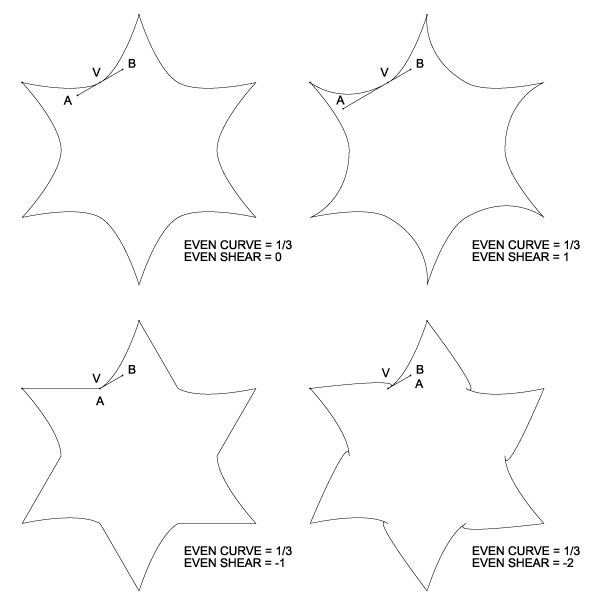### Even Shear

Even Shear makes the curvature at even vertices asymmetrical. Curve asymmetry at odd vertices is controlled separately, via the Odd Shear parameter. The two curve control points at each vertex are normally contrained to be equidistant from the vertex. Removing this constraint yields two distinct distances, one between the counterclockwise point (A) and the vertex, and the other between the clockwise point (B) and the vertex. Shear changes the ratio of these two distances. Shear is normalized so that at zero, the control points are equidistant as usual, while at −1, the counterclockwise point coincides with the vertex, eliminating curvature on that side. Note that for Even Shear to have any effect, Even Curve must have a nonzero value.